## Elliptic Integral Singular Value

When the Modulushas a singular value, the complete elliptic integrals may be computed in analytic form in terms of Gamma Functions. Abel(quoted in Whittaker and Watson 1990, p. 525) proved that whenever(1)

where,,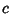,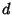, andare Integers,is a complete Elliptic Integral of the First Kind, andis the complementary complete Elliptic Integral of the First Kind, then the Modulusis the Root of an algebraic equation with Integer Coefficients.

A Modulus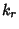such that(2)

is called a singular value of the elliptic integral. The Elliptic Lambda Functiongives the value of. Selberg and Chowla (1967) showed that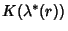andare expressible in terms of a finite number of Gamma Functions. The complete Elliptic Integrals of the Second Kindand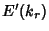can be expressed in terms ofand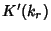with the aid of the Elliptic Alpha Function.

The following table gives the values offor small integral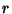in terms of Gamma Functions.where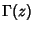is the Gamma Function andis an algebraic number (Borwein and Borwein 1987, p. 298).

Borwein and Zucker (1992) give amazing expressions for singular values of complete elliptic integrals in terms of Central Beta Functions(3)

Furthermore, they show that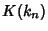is always expressible in terms of these functions for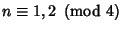. In such cases, the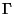functions appearing in the expression are of the form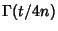whereand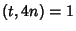. The terms in the numerator depend on the sign of the Kronecker Symbol. Values for the first feware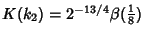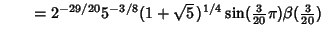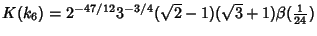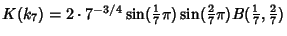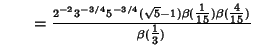where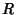is the Real Root of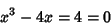(4)

and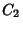is an algebraic number (Borwein and Zucker 1992). Note thatis the only value in the above list which cannot be expressed in terms of Central Beta Functions.

Using the Elliptic Alpha Function, the Elliptic Integrals of the Second Kind can also be found from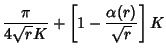(5)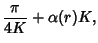(6)

and by definition,(7)

See also Central Beta Function, Elliptic Alpha Function, Elliptic Delta Function, Elliptic Integral of the First Kind, Elliptic Integral of the Second Kind, Elliptic Lambda Function, Gamma Function, Modulus (Elliptic Integral)

References

Abel, N. H. Recherches sur les fonctions elliptiques.'' J. reine angew. Math. 3, 160-190, 1828. Reprinted in Abel, N. H. Oeuvres Completes (Ed. L. Sylow and S. Lie). New York: Johnson Reprint Corp., p. 377, 1988.

Borwein, J. M. and Borwein, P. B. Pi & the AGM: A Study in Analytic Number Theory and Computational Complexity. New York: Wiley, pp. 139 and 298, 1987.

Borwein, J. M. and Zucker, I. J. Elliptic Integral Evaluation of the Gamma Function at Rational Values of Small Denominator.'' IMA J. Numerical Analysis 12, 519-526, 1992.

Bowman, F. Introduction to Elliptic Functions, with Applications. New York: Dover, pp. 75, 95, and 98, 1961.

Glasser, M. L. and Wood, V. E. A Closed Form Evaluation of the Elliptic Integral.'' Math. Comput. 22, 535-536, 1971.

Selberg, A. and Chowla, S. On Epstein's Zeta-Function.'' J. Reine. Angew. Math. 227, 86-110, 1967.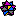Weisstein, E. W. Elliptic Singular Values.'' Mathematica notebook EllipticSingular.m.

Whittaker, E. T. and Watson, G. N. A Course in Modern Analysis, 4th ed. Cambridge, England: Cambridge University Press, pp. 524-528, 1990.

Wrigge, S. An Elliptic Integral Identity.'' Math. Comput. 27, 837-840, 1973.

Zucker, I. J. The Evaluation in Terms of-Functions of the Periods of Elliptic Curves Admitting Complex Multiplication.'' Math. Proc. Cambridge Phil. Soc. 82, 111-118, 1977.